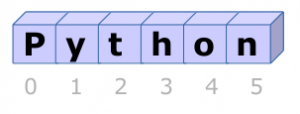# Python stringsa string, series of characters

A string is a series of characters, they are mostly used to display text.

To define a string simply type text between quotes. Python accepts single, double and triple quotes.

Related Course:
Python Programming Bootcamp: Go from zero to hero

## String input and output

To output text (string) to the screen:

To get text from keyboard:

If you use an old Python version (2.x), you need to use:

python –version

## String Comparison

To test if two strings are equal use the equality operator (==).

To test if two strings are not equal use the inequality operator (!=)

## 52 thoughts on “Python strings”

1.- December 10, 2015

Why do I get syntax error, when I use the( ==) operator to compare equalities. For example if x = 3 and y = 3, when I press enter, I get a syntax error. Please tell me where I am going wrong.

1.- December 10, 2015

Are you running the code or using the interpreter directly?
Do you see >>> before the text you type? If so, you have to create a file with the extension .py and execute with that as parameter.

2.- September 21, 2015

am much interested to learn programming,,am going through tutorials but is possible to get practical tutorials

3.- September 11, 2015

You have spelled “concatenation” wrongly: the word is “concatenate” — note the ‘e’ in the middle — NOT “concatinate”. (It means ‘link together like a chain’ because it comes from the Latin ‘catena’ = chain.)

1.- September 11, 2015

Thanks Jem, I updated it.

4.- September 2, 2015

sir? can you explain the equality operations? i don’t get it……

1.- September 2, 2015

Hi,

The equality operator (==) tests if two variables have an equal value. Given variable x=3 and y=3, we can test for equality using the statement if x == y, which will return true. If we change either x or y, it would return false. It looks like the assignment operator (=) , but the purpose of the assignment operator is only to set the variables data. You can use the operator on strings, integers, decimals and many other types of variables. To test for inequality use the not operator (!=).

1.- September 3, 2015

oh i get it now thanks alot sir